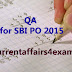Sunday, May 31, 2015

# Quantitative Aptitude Quiz for SBI PO 2015

1) If cost price of 30 pens is equal to selling price of 24 pens, profit percentage is?
(A) 10%
(B) 20%
(C) 25%
(D) 16%
(E) None of these

2)  In an election between two candidates one who got 35% of the votes lost the election by 720 votes. Then total votes polled in the election was?
(A) 1800
(B) 2400
(C) 3200
(D) 3800
(E) None of these

3) A number when divided by 91 gives a reminder 17. When the same number is divided by 13 the reminder will be?
(A)  2
(B) 4
(C) 5
(D) 1
(E) None of these

4) 25 litres of salt solution contains 6% salt. How many litres of water must he added so as to get a resultant solution containing 5% salt?
(A) 5 litres
(B) 4 litres
(C) 8 litres
(D) 6 litres
(E) None of these

5) The difference between simple interest and compound interest on a sum of money at 5% per annum for 2 years is Rs.100. The sum of the money is?
(A) 50000
(B) 42500
(C) 40000
(D) 25000
(E) None of these

6) In how many years a sum of money doubles in simple interest if rate of interest is 12%?
(A) 8 Years
(B) 12 years
(C) 8 & 1/3 years
(D) 14 years
(E) None of these

7) The average of 17 numbers is 45. The average of first 9 of these numbers is 51 and last 9 of these numbers is 36. What is the ninth number?
(A) 16
(B) 18
(C) 15
(D) 17
(D) None of these

8) The price of an article is cut by 10%. To restore it to the former price the new price must be increased by?
(A) 11%
(B) 10%
(C) 11 &1/9%
(D) 10 & 1/9%
(E) None of these

9)  By Selling 66 pens one gain selling price of 22 pens. Gain percentage is
(A) 20%
(B) 40%
(C) 25%
(D) 50%
(E) None of these

10)  If the length of a rectangle is increased by 30%, its breadth is increased by 20%. Its area will be increased by?
(A) 50%
(B) 53%
(C) 52%
(D) 56%
(E)None of these

1) Ans. (C) 25%
Solution: If cost price of x articles is equal to selling price of y articles, then
Profit% = ((x-y/y)*100) %
2) Ans. (B) 2400
3) Ans. (B) 4
4) Ans. (A) 5 litres
5) Ans. (C) 40000
Solution: Formulae for difference between simple interest and compound interest = PR^2/10000
6) Ans. (C) 8 & 1/3 years
7) Ans. (B) 18
8) Ans. (C) 11 &1/9%
9) Ans. (D) 50%
10) Ans. (D) 56%
Quantitative Aptitude Quiz for SBI PO 2015Reviewed by Currentaffairs4examz on 31.5.15 Rating: 5 1) If cost price of 30 pens is equal to selling price of 24 pens, profit percentage is? (A) 10% (B) 20% (C) 25% (D) 16% (E) None...